• Excel中有很多执行线性代数运算的函数，只要轻点鼠标，各种计算都手到擒来。这部分，我们将介绍矩阵求逆的方法。
Excel中有很多执行线性代数运算的函数，只要轻点鼠标，各种计算都手到擒来。这一部分，我们将介绍矩阵求逆的方法。对于n阶方阵A，如果另外有一个n阶方矩阵B，使得A×B=E，E为单位矩阵，则A和B互为逆矩阵。比如下面的例子，读者可以利用手把手教你用Execel计算两个矩阵的乘法中提供的方法来验证它们相乘的结果是一个单位矩阵。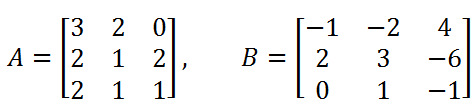注意根据线性代数的知识：（1）只有方阵才有逆矩阵；（2）A是可逆矩阵的充分必要条件是矩阵的行列式∣A∣≠0，即可逆矩阵就是非奇异矩阵。首先，我们在EXCEL中输入一个待求逆的矩阵，如下图所示，其中蓝色部分表示A矩阵。然后再选定一个与A同样大小的区域作为显示计算结果的部分，如图中黄色部分所示。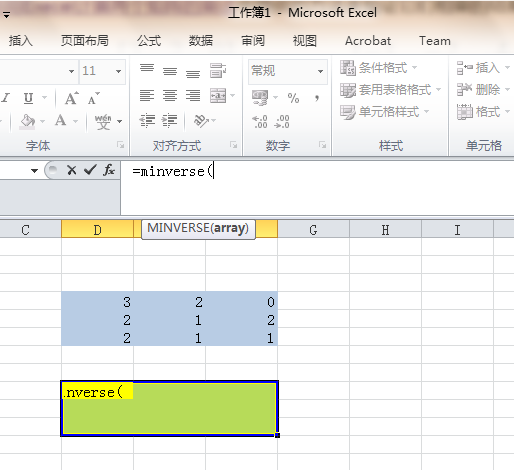EXCEL中提供的矩阵求逆函数是MINVERSE，所以在选定显示计算结果的区域之后，我们就可以在公式栏中编辑公式，如果你看不到公示栏，那么先输入等号“=”，EXCEL就会知道你将要输入的是一个公式。然后在函数MINVERSE的参数部分，选定蓝色的区域，如下所示。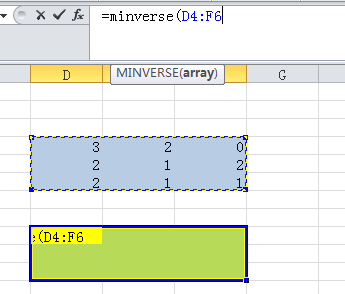主要要把括号输入完整。然后，同时按下Ctrl + Shift + Enter， 就大功告成啦！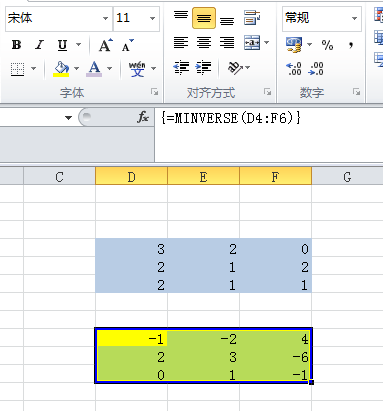展开全文数学 Excel 线性代数
• numpy中创建单位矩阵借助identity（）函数。更为准确的说，此函数创建的是一个n*n的单位数组，返回值的dtype=array数据形式。其中接受的参数有两个，第一个是n值大小，第二个为数据类型，一般为浮点型。单位数组的...
在学习linear regression时经常处理的数据一般多是矩阵或者n维向量的数据形式，所以必须对矩阵有一定的认识基础。
numpy中创建单位矩阵借助identity（）函数。更为准确的说，此函数创建的是一个n*n的单位数组，返回值的dtype=array数据形式。其中接受的参数有两个，第一个是n值大小，第二个为数据类型，一般为浮点型。单位数组的概念与单位矩阵相同，主对角线元素为1，其他元素均为零，等同于单位1。而要想得到单位矩阵，只要用mat（）函数将数组转换为矩阵即可。
>>> import numpy as np
>>> help(np.identity)

Help on function identity in module numpy:

identity(n, dtype=None)
Return the identity array.

The identity array is a square array with ones on
the main diagonal.

Parameters
----------
n : int
Number of rows (and columns) in n x n output.
dtype : data-type, optional
Data-type of the output.  Defaults to float.

Returns
-------
out : ndarray
n x n array with its main diagonal set to one,
and all other elements 0.

Examples
--------
>>> np.identity(3)
array([[ 1.,  0.,  0.],
[ 0.,  1.,  0.],
[ 0.,  0.,  1.]])
>>> np.identity(5)

array([[1., 0., 0., 0., 0.],
[0., 1., 0., 0., 0.],
[0., 0., 1., 0., 0.],
[0., 0., 0., 1., 0.],
[0., 0., 0., 0., 1.]])
>>> A = np.mat(np.identity(5))

>>> A

matrix([[1., 0., 0., 0., 0.],
[0., 1., 0., 0., 0.],
[0., 0., 1., 0., 0.],
[0., 0., 0., 1., 0.],
[0., 0., 0., 0., 1.]])

矩阵的运算中还经常使用对角阵，numpy中的对角阵用eye（）函数来创建。eye（）函数接受五个参数，返回一个单位数组。第一个和第二个参数N，M分别对应表示创建数组的行数和列数，当然当你只设定一个值时，就默认了N=M。第三个参数k是对角线指数，跟diagonal中的offset参数是一样的，默认值为0，就是主对角线的方向，上三角方向为正，下三角方向为负，可以取-n到+m的范围。第四个参数是dtype，用于指定元素的数据类型，第五个参数是order，用于排序，有‘C’和‘F’两个参数，默认值为‘C’，为行排序，‘F’为列排序。返回值为一个单位数组。
>>> help(np.eye)

Help on function eye in module numpy:

eye(N, M=None, k=0, dtype=<class 'float'>, order='C')
Return a 2-D array with ones on the diagonal and zeros elsewhere.

Parameters
----------
N : int
Number of rows in the output.
M : int, optional
Number of columns in the output. If None, defaults to N.
k : int, optional
Index of the diagonal: 0 (the default) refers to the main diagonal,
a positive value refers to an upper diagonal, and a negative value
to a lower diagonal.
dtype : data-type, optional
Data-type of the returned array.
order : {'C', 'F'}, optional
Whether the output should be stored in row-major (C-style) or
column-major (Fortran-style) order in memory.

Returns
-------
I : ndarray of shape (N,M)
An array where all elements are equal to zero, except for the k-th
diagonal, whose values are equal to one.

--------
identity : (almost) equivalent function
diag : diagonal 2-D array from a 1-D array specified by the user.

Examples
--------
>>> np.eye(2, dtype=int)
array([[1, 0],
[0, 1]])
>>> np.eye(3, k=1)
array([[ 0.,  1.,  0.],
[ 0.,  0.,  1.],
[ 0.,  0.,  0.]])

numpy中的diagonal（）方法可以对n*n的数组和方阵取对角线上的元素，diagonal（）接受三个参数。第一个offset参数是主对角线的方向，默认值为0是主对角线，上三角方向为正，下三角方向为负，可以取-n到+n的范围。第二个参数和第三个参数是在数组大于2维时指定一个2维数组时使用，默认值axis1=0，axis2=1。
>>> help(A.diagonal)

Help on built-in function diagonal:

diagonal(...) method of numpy.matrix instance
a.diagonal(offset=0, axis1=0, axis2=1)

Return specified diagonals. In NumPy 1.9 the returned array is a
read-only view instead of a copy as in previous NumPy versions.  In
a future version the read-only restriction will be removed.

Refer to :func:numpy.diagonal for full documentation.

--------
numpy.diagonal : equivalent function
>>> help(np.diagonal)

Help on function diagonal in module numpy:

diagonal(a, offset=0, axis1=0, axis2=1)
Return specified diagonals.

If a is 2-D, returns the diagonal of a with the given offset,
i.e., the collection of elements of the form a[i, i+offset].  If
a has more than two dimensions, then the axes specified by axis1
and axis2 are used to determine the 2-D sub-array whose diagonal is
returned.  The shape of the resulting array can be determined by
removing axis1 and axis2 and appending an index to the right equal
to the size of the resulting diagonals.

In versions of NumPy prior to 1.7, this function always returned a new,
independent array containing a copy of the values in the diagonal.

In NumPy 1.7 and 1.8, it continues to return a copy of the diagonal,
but depending on this fact is deprecated. Writing to the resulting
array continues to work as it used to, but a FutureWarning is issued.

Starting in NumPy 1.9 it returns a read-only view on the original array.
Attempting to write to the resulting array will produce an error.

In some future release, it will return a read/write view and writing to
the returned array will alter your original array.  The returned array
will have the same type as the input array.

If you don't write to the array returned by this function, then you can
just ignore all of the above.

If you depend on the current behavior, then we suggest copying the
returned array explicitly, i.e., use np.diagonal(a).copy() instead
of just np.diagonal(a). This will work with both past and future
versions of NumPy.

Parameters
----------
a : array_like
Array from which the diagonals are taken.
offset : int, optional
Offset of the diagonal from the main diagonal.  Can be positive or
negative.  Defaults to main diagonal (0).
axis1 : int, optional
Axis to be used as the first axis of the 2-D sub-arrays from which
the diagonals should be taken.  Defaults to first axis (0).
axis2 : int, optional
Axis to be used as the second axis of the 2-D sub-arrays from
which the diagonals should be taken. Defaults to second axis (1).

Returns
-------
array_of_diagonals : ndarray
If a is 2-D, then a 1-D array containing the diagonal and of the
same type as a is returned unless a is a matrix, in which case
a 1-D array rather than a (2-D) matrix is returned in order to
maintain backward compatibility.

If a.ndim > 2, then the dimensions specified by axis1 and axis2
are removed, and a new axis inserted at the end corresponding to the
diagonal.

Raises
------
ValueError
If the dimension of a is less than 2.

--------
diag : MATLAB work-a-like for 1-D and 2-D arrays.
diagflat : Create diagonal arrays.
trace : Sum along diagonals.

Examples
--------
>>> a = np.arange(4).reshape(2,2)
>>> a
array([[0, 1],
[2, 3]])
>>> a.diagonal()
array([0, 3])
>>> a.diagonal(1)
array()

A 3-D example:

>>> a = np.arange(8).reshape(2,2,2); a
array([[[0, 1],
[2, 3]],
[[4, 5],
[6, 7]]])
>>> a.diagonal(0, # Main diagonals of two arrays created by skipping
...            0, # across the outer(left)-most axis last and
...            1) # the "middle" (row) axis first.
array([[0, 6],
[1, 7]])

The sub-arrays whose main diagonals we just obtained; note that each
corresponds to fixing the right-most (column) axis, and that the
diagonals are "packed" in rows.

>>> a[:,:,0] # main diagonal is [0 6]
array([[0, 2],
[4, 6]])
>>> a[:,:,1] # main diagonal is [1 7]
array([[1, 3],
[5, 7]])
>>> A = np.random.randint(low=5, high=30, size=(5, 5))

>>> A

array([[25, 15, 26,  6, 22],
[27, 14, 22, 16, 21],
[22, 17, 10, 14, 25],
[11,  9, 27, 20,  6],
[24, 19, 19, 26, 14]])
>>> A.diagonal()

array([25, 14, 10, 20, 14])
>>> A.diagonal(offset=1)

array([15, 22, 14,  6])
>>> A.diagonal(offset=-2)

array([22,  9, 19])



展开全文• ## 矩阵

千次阅读 2015-06-29 11:43:57
m行n列矩阵A，记作 。...有元素为复数则叫 复矩阵· 行数和列数都等于n的矩阵，叫n阶矩阵 或 n阶方阵： An· 只有行的矩阵A=(a1, a2, ..., an) 称为 行矩阵、行向量· 只有列的矩阵 称为 列矩阵、列...
m行n列矩阵A，记作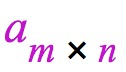。 如下图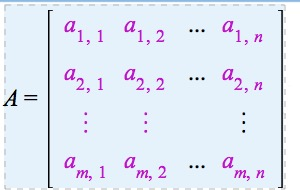下标间的 逗号可以不要数aij(ij为下标)位于矩阵A的 i行j列，称为矩阵A的(i,j)位置的元素(简称为元)， 记作 (aij)或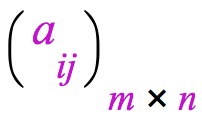· 元素为实数叫 实矩阵。有元素为复数则叫 复矩阵· 行数和列数都等于n的矩阵，叫n阶矩阵 或 n阶方阵： An· 只有一行的矩阵A=(a1, a2, ..., an) 称为 行矩阵、行向量· 只有一列的矩阵 称为 列矩阵、列向量· 若两个矩阵行列相等，即称为 同型矩阵       A=(aij)，B=(bij)，若aij=bij (i=1,2,...,m;j=1,2,...,n)  => A=B· 元素都为零的 称作 零矩阵  记作0.  注意不同型的0矩阵是不同的·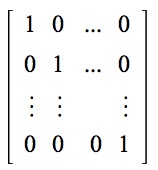像这样从左上到右下对角线(即主对角线)上的元素为1，其它都为0的方阵，称作单位矩阵 E   单位矩阵中的元素aij={1, 当i=j；0,当i≠j}· 不在主对角线上的元素都为0（对角线上的元素为任意值），这样的方阵称为 对角矩阵     A=diag(a1,a2,...,an)· 主对角元素都相等的对角矩阵，称为 数量矩阵，也称纯量阵· aij=aji 的方阵，称为 对称矩阵  如：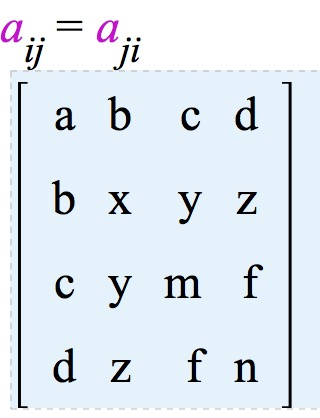· aij=-aji 的方阵， 称为 反对称矩阵 如：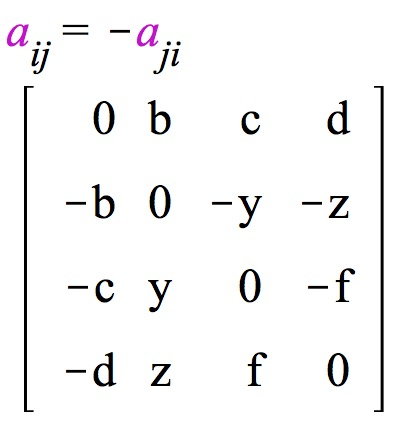反对称矩阵的 主对角线上的 元素 必须是 0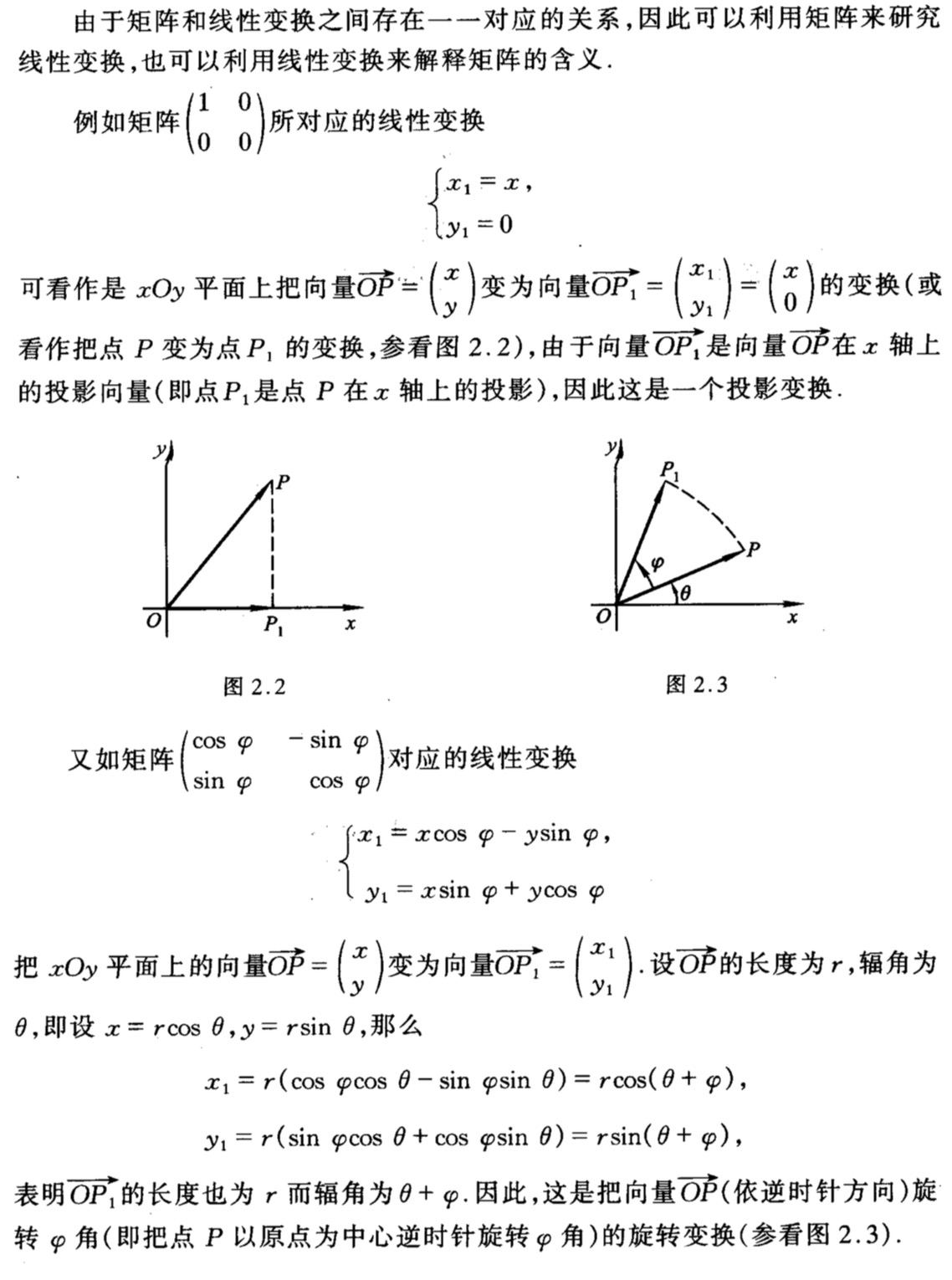用代码验证了下：int a = 33, b = 38;//角度
double r = 10; //op长度

double acos = Math.cos(Math.PI/180 * a);
double bcos = Math.cos(Math.PI/180 * b);
double asin = Math.sin(Math.PI/180 * a);
double bsin = Math.sin(Math.PI/180 * b);

//x=
System.out.println((acos*bcos - asin * bsin) * r);
System.out.println(Math.cos(Math.PI / 180 * (a + b)) * r);
//y=
System.out.println((acos*bsin + asin * bcos) * r );
System.out.println(Math.sin(Math.PI / 180 * (a + b)) * r);逆矩阵若AB=BA=单位矩阵，则AB互为逆矩阵。AB都是n阶矩阵逆矩阵的应用：若AB互为逆矩阵， 且EA=C；则:EAB=CB；E=CB所以在已知结果矩阵C和原矩阵A，求E矩阵时，只需要用C乘以A的逆矩阵B矩阵的运算· 加法。 同型矩阵 才能相加。 A+B=B+A。运算时：(aij)+(bij)。  A-B = A+(-B)。A+(-A)=0 （0型矩阵）· 矩阵与数相乘。 运算时：λ*A =λ乘以A中的每一项(aij)。    若有数x、y，矩阵A、B：(xy)A=x(yA)；(x+y)A=xA+yA；x(A+B)=xA+xB  矩阵相加与数乘矩阵 统称为 矩阵的线性运算· 矩阵乘矩阵。AB相乘，乘号左边矩阵的列要等于右边矩阵的行(A列等于B行)，才能相乘; 而结果为A行B列  乘积矩阵AB=C的(i,j)元cij就是A的i行与B的j列的乘积之和，且A的每一行要乘以B的每一列如：A的i行 为 {1, 2, 3} ; B的j列 为 {4, 5, 6}  ==> {1x4 + 2x5 + 3x6}  (AB)C=A(BC);  k(AB)=(kA)B=A(kB); (k为数)  A(B+C)=AB+AC，(B+C)A=BA+CA;  AB≠BA；  乘单位矩阵E：AE=EA=A  kE即是纯量阵。(kE)A=k(EA)=kA，A(kE)=AkE=kAE=kA      若A为n阶方阵时， (kE)A=kA=A(kE)； 这时可以交换。因AE都是n阶方阵，且E为纯量阵，所以只有第ixi可以相乘，这时AE=EA
展开全文• 线性代数提供了被称为逆矩阵（matrix inversion）的强大工具。对于大多数矩阵A，我们都能通过矩阵逆解析地求解式Ax=bAx=b。
　　线性代数提供了被称为逆矩阵（matrix inversion）的强大工具。对于大多数矩阵A，我们都能通过矩阵逆解析地求解式Ax=b<!--//--><![CDATA[//><!--
Ax=b
//--><!]]>。

8.3.1 单位矩阵

为了描述矩阵逆，首先需要定义单位矩阵（identity matrix）的概念。任意向量和单位矩阵相乘，都不会改变。我们将保持n<!--//--><![CDATA[//><!--
n
//--><!]]>维向量不变的单位矩阵记作In<!--//--><![CDATA[//><!--
I_n
//--><!]]>。形式上，In∈Rn×n<!--//--><![CDATA[//><!--
I_n\in R^{n\times n}
//--><!]]>， ∀x∈Rn,Inx=x(8.20)<!--//--><![CDATA[//><!--
//--><!]]>　　单位矩阵的结构很简单：
1. 它是“正方形”（行数与列数相同）
2. 所有沿主对角线的元素都是1，而所有其他位置的元素都是0 ⎡⎣⎢100010001⎤⎦⎥<!--//--><![CDATA[//><!--
\begin{bmatrix} 1&0&0 \\ 0&1&0 \\0&0&1 \end{bmatrix}
//--><!]]>8.3.2 逆矩阵概念

矩阵A<!--//--><![CDATA[//><!--
A
//--><!]]>的逆矩阵（matrix inversion）记作A−1<!--//--><![CDATA[//><!--
A^{-1}
//--><!]]>，其定义的矩阵满足如下条件： A−1A=In(8.21)<!--//--><![CDATA[//><!--
//--><!]]>
其实矩阵的逆矩阵跟倒数的性质一样，不过只是我们习惯用A−1<!--//--><![CDATA[//><!--
A^{-1}
//--><!]]>表示。而倒数可以表示成1/x<!--//--><![CDATA[//><!--
1/x
//--><!]]>或者x−1<!--//--><![CDATA[//><!--
x^{-1}
//--><!]]>的形式，而不能把A<!--//--><![CDATA[//><!--
A
//--><!]]>的逆矩阵写成1/A<!--//--><![CDATA[//><!--
1/A
//--><!]]>的形式，其主要原因是矩阵不能被除。
为了理解矩阵的逆，其和倒数还有其他相似之处：

当我们将一个数乘以它的倒数我们得1<!--//--><![CDATA[//><!--
1
//--><!]]>。1×(1/8)=1<!--//--><![CDATA[//><!--
1 \times (1/8) = 1
//--><!]]>
当一个矩阵乘以逆时，我们得到了单位矩阵。A×A−1=I<!--//--><![CDATA[//><!--
A \times A^{-1}=I
//--><!]]>
矩阵与逆矩阵乘法与数乘一样，交换位置结果不变(1/8)×8=1<!--//--><![CDATA[//><!--
(1/8) \times 8 = 1
//--><!]]>，即乘法满足交换律A−1×A=I<!--//--><![CDATA[//><!--
A^{-1}\times A= I
//--><!]]>
8.3.3 行列式和求解逆矩阵

{\begin{matrix}
\begin{bmatrix}
a&b \\
c&d
\end{bmatrix}^{-1}=
\begin{bmatrix}
d&-b \\
-c&a
\end{bmatrix}
\end{matrix}}
//--><!]]>
{\begin{matrix}
det \begin{bmatrix}
a&b \\
c&d
\end{bmatrix}
\neq 0
\Leftrightarrow
\begin{bmatrix}
a&b \\
c&d
\end{bmatrix}
^{-1}
\end{matrix}}
//--><!]]>　　例题：利用逆矩阵的概念逆推，即将矩阵乘以逆矩阵，最终求得单位矩阵。8.3.4 求解矩阵方程

矩阵中没有被除的概念，而矩阵的逆，可以解决“矩阵除法”的问题。假如我们没有“除法”规则，那么解决“把10个苹果分给两个人”的问题，可以采取2的倒数（12=0.5<!--//--><![CDATA[//><!--
\frac{1}{2}=0.5
//--><!]]>）来计算，那么答案就是10×0.5=5<!--//--><![CDATA[//><!--
10 \times 0.5 = 5
//--><!]]>，也就是每个人5个苹果。
我们也可以利用以上方法，已知矩阵A<!--//--><![CDATA[//><!--
A
//--><!]]>和矩阵B<!--//--><![CDATA[//><!--
B
//--><!]]>，求解矩阵X<!--//--><![CDATA[//><!--
X
//--><!]]>。即XA=B<!--//--><![CDATA[//><!--
XA = B
//--><!]]>。最好的方法是直接除以A<!--//--><![CDATA[//><!--
A
//--><!]]>，得到X=B/A<!--//--><![CDATA[//><!--
X=B/A
//--><!]]>，但事实上我们不能直接除以A<!--//--><![CDATA[//><!--
A
//--><!]]>。但我们可以在公式两边都乘以A−1<!--//--><![CDATA[//><!--
A^{-1}
//--><!]]>。即XAA−1=BA−1<!--//--><![CDATA[//><!--
XAA^{-1}=BA^{-1}
//--><!]]>。
因为AA−1=I<!--//--><![CDATA[//><!--
AA^{-1}= I
//--><!]]>，所以就得到XI=BA−1<!--//--><![CDATA[//><!--
XI=BA^{-1}
//--><!]]>。而此时单位矩阵I<!--//--><![CDATA[//><!--
I
//--><!]]>可以直接去掉，于是求得X=BA−1<!--//--><![CDATA[//><!--
X=BA^{-1}
//--><!]]>。因此通过A−1<!--//--><![CDATA[//><!--
A^{-1}
//--><!]]>，就可以直接计算出矩阵X<!--//--><![CDATA[//><!--
X
//--><!]]>。

例题：
有一个几个家庭组团出去旅行，出发的时候是乘坐大巴，每位儿童3元，每个大人3.2元，一共花费了118.4元。在回程时，他们选择乘坐火车，每名儿童3.5元，每名成人3.6元，总计135.20元。求解有多少儿童和大人？

我们尝试用矩阵思维来解答，首先设置好矩阵（注意矩阵的行和列是否正确）：然后求解A<!--//--><![CDATA[//><!--
A
//--><!]]>的逆矩阵：根据公式X=BA−1<!--//--><![CDATA[//><!--
X=BA^{-1}
//--><!]]>，求解X<!--//--><![CDATA[//><!--
X
//--><!]]>。根据求解所得，一共有16<!--//--><![CDATA[//><!--
16
//--><!]]>个儿童和22<!--//--><![CDATA[//><!--
22
//--><!]]>个大人。这样的计算其实非常有利于工程师设计建筑物，视频游戏和计算机动画等许多地方，它是解线性方程组的一种解法。虽然是求矩阵的逆，只要打开Python工具的NumPy库，输入numpy.inv(A)，即可求得A<!--//--><![CDATA[//><!--
A
//--><!]]>的逆矩阵。虽然这个过程是由计算机完成，但我们还是有必要去了解公式，因为这正是数学的美妙之处。
展开全文• * 使用单位矩阵拼接的形式 * @author imlilu * */ public class InverseMatrixSecond { /*** * 增广矩阵机型初等行变化的算法 * * @param value * 需要算的增广矩阵 * @return 计算的结java 算法
• 、0矩阵 二、1矩阵 三、单位矩阵 四、深拷贝和浅拷贝 五、创建等差数组 — 指定数量 六、创建等差数组 — 指定步长 七、创建等比数列
• 参考链接： ...s_tid=doc_srchtitle 已知矩阵A求F(t)=e^At状态转移矩阵 方法： 使用到的函数为expm 矩阵指数 语法 Y = expm(X) 说明 Y = expm (X) 计算 X 的...Matlab
• 关于正定矩阵的一些运算正交矩阵矩阵的特征...EEE 是单位矩阵 矩阵的特征分解 对于一个矩阵其特征值来说，有以下基本条件： Av=λvAv =\lambda vAv=λv 同样我们可以得到矩阵的特征值分解 A=QΣQ−1A=Q\Sigma Q^{-1...数学
• 设矩阵A满足A2+A−4E=0A^2+A-4E = 0,其中E单位矩阵，则(A+E)−1=?(A+E)^{-1} = ? 分析：这种抽象矩阵的逆矩阵的求法只能用定义式了，但是怎么拆出需要的定义式呢？分享种绝对适用的解法。如果我说，眼看出来...
• 提到案例分析就头疼的小伙伴们，分享BCG的经典波士顿矩阵，再也不怕案例分析啦！制定公司层战略最流行的方法之就是BCG矩阵。该方法是由波士顿咨询集团（Boston Co...
• ## MATLAB01:基本的数学运算与矩阵运算

万次阅读 多人点赞 2019-11-12 17:22:52
MATLAB01:基本的数学运算与矩阵运算MATLAB基本语法变量变量名保留变量不适合做变量名变量不应当覆盖内置函数MATLAB的调用优先级变量类型数字型变量的显示格式MATLAB命令行使用MATLAB进行数字运算使用MATLAB计算数学...MATLAB MATLAB变量
• http://www.cnblogs.com/JoeDZ/archive/2008/06/30/1232741.html #ifndef _MATRIX_H #define _MATRIX_H class Matrix { private: int row; // 矩阵的行数 int col; // 矩阵的列数matrix delete ios c
• 单应矩阵H本征矩阵E对极几何在双目问题中非常的重要，可以简化立体匹配等问题，而要应用对极几何去解决问题，比如求极线，需要知道本征矩阵或者基础矩阵，因此双目标定过程中也会把本征矩阵和基础矩阵算出来。...立体视觉 计算机视觉 三维测量
• ## 矩阵的逆

万次阅读 2021-03-29 10:03:25
将原矩阵A和一个单位矩阵E作成大矩阵(A, E)，用初等行变换将大矩阵中的A变为E，则会得到(E, A-1)的形式。 时间复杂度：O(n3) inline vector<double> operator * (vector<double> a, double b) { int N ...线性代数 数据结构 c++
• ## 矩阵乘以其矩阵转置

万次阅读 多人点赞 2018-08-27 20:47:11
A∗ATA∗ATA*A^T 得到一个 m∗mm∗mm*m 矩阵，AT∗AAT∗AA^T*A 得到一个 n∗nn∗nn*n 的矩阵，这样我们就能得到一个矩阵。 看一个例子: Xθ=HXθ=HX \theta =H 求解θθ\theta. XTXθ=XTHXTXθ=XT...机器学习 数学
• ## 酉矩阵

万次阅读 多人点赞 2019-10-23 20:17:12
1.酉矩阵（unitary matrix）若n阶复矩阵A满足 AHA=AAH=E AHA=AAH=E ...(4)充分条件是它的n列向量是两两正交的单位向量。 该部分参考这篇博客link 共轭转置 首先将A中的每元素aij取共轭得bij，将...线性代数3d shader
• 一个矩阵M, 把它的第一行变成第一列，第二行变成第二列等，最末一行变为最末一列， 从而得到一个新的矩阵N。 这一过程称为矩阵的转置。即矩阵A的行和列对应互换。 （2）逆矩阵的含义：一个n阶方阵A称为可逆的，
• ## OpenCV—基本矩阵操作与示例

万次阅读 多人点赞 2016-05-02 16:30:14
图像处理中对矩阵的操作非常重要，本文总结了使用OpenCV进行矩阵的创建、初始化以及基本矩阵操作，给出了示例代码。OpenCV C++ 图像处理
• 再介绍奇异矩阵以及矩阵的广义逆之前，先复习几概念，然后我步步给出奇异矩阵和广义逆的介绍。 伴随矩阵 1. 余子式 定义1 余子式：在nnn阶行列式|A||A||A|中，划去元素aijaija_{ij}所在的行和列，剩下的...代数余子式
• 1. 矩阵和向量1.1 矩阵⎡⎣⎢⎢⎢⎢1402137194914719182114371448⎤⎦⎥⎥⎥⎥ \begin{bmatrix} ...这是4x2的矩阵，即4行2列。矩阵的维度即行数乘以列数。矩阵的元素（矩阵项）： A=⎡⎣⎢⎢⎢⎢机器学习
• 这一节Jungle用C++设计了矩阵的类Matrix，并设计了3方法： 矩阵相加add 矩阵相乘multiply 求矩阵的逆矩阵inverse 有了这三方法，足以进行机器人正逆运动学分析了。 直接上代码： #include&amp;amp;amp;lt;...
• 设A和E是同阶矩阵，则AE=EA=A 下面的证明只是简单的使用了矩阵乘法的定义。
• ## 矩阵的初等变换

千次阅读 2016-10-13 09:26:31
Ei(k)E_i(k)：单位矩阵的第i行或者第i列乘以k倍得到的矩阵。 EijE_{ij}：单位矩阵第i行和第j行交换或者第i列和第j列交换得到的矩阵。 Eij(k)E_{ij}(k)：单位矩阵的第j行乘以k倍加到第i行，即被操作的行在前；那么...初等变换
• 今天复习数值分析在对矩阵进行Doolittle分解时发现了一些有意思的事情，请容我慢慢道来… 正文 先介绍矩阵得三角分解即Doolittle分解定理： 定理 ：A∈Rn×nA\in R^{n\times n}A∈Rn×n，若 detA≠0,Δi≠0(i=1,2,...
• ## 矩阵分解

千次阅读 2019-04-12 21:59:27
所谓矩阵分解就是将矩阵分解成两三标准矩阵的乘积 矩阵分解的目的在于 求解线性方程组 矩阵存储 矩阵重构以预测 矩阵分解的根本方法在于线性变换 矩阵分解的分类 对角化分解：通过正交变换将矩阵对角化 奇异...数值线性代数
• 当我们用matlab计算矩阵和逆矩阵时，结果往往不等于单位阵 如下： &gt;&gt; a=magic(4) a =  16 2 3 13  5 11 10 8  9 7 6 12  4 14 15 1 &gt;&gt; b=a*inv(a) 警告: 矩阵接近奇异值，或者...matlab
• 本篇继续参照高翔老师《视觉SLAM十四讲从理论到实践》，讲解三维...本文相对于原文会适当精简，同时为便于理解，会加入一些注解和补充知识点，本篇为第部分：旋转矩阵和变换矩阵，另外三部分请参照博主的其他博文。线性代数...